Electric Circuits - Lesson 1 - Electric Potential Difference

# Electric PotentialIn the previous section of Lesson 1, it was reasoned that the movement of a positive test charge within an electric field is accompanied by changes in potential energy. A gravitational analogy was relied upon to explain the reasoning behind the relationship between location and potential energy. Moving a positive test charge against the direction of an electric field is like moving a mass upward within Earth's gravitational field. Both movements would be like going against nature and would require work by an external force. This work would in turn increase the potential energy of the object. On the other hand, the movement of a positive test charge in the direction of an electric field would be like a mass falling downward within Earth's gravitational field. Both movements would be like going with nature and would occur without the need of work by an external force. This motion would result in the loss of potential energy. Potential energy is the stored energy of position of an object and it is related to the location of the object within a field. In this section of Lesson 1, we will introduce the concept of electric potential and relate this concept to the potential energy of a positive test charge at various locations within an electric field.

### The Gravitational Analogy Revisited

A gravitational field exists about the Earth that exerts gravitational influences upon all masses located in the space surrounding it. Moving an object upward against the gravitational field increases its gravitational potential energy. An object moving downward within the gravitational field would lose gravitational potential energy. When gravitational potential energy was introduced in Unit 5 of The Physics Classroom, it was defined as the energy stored in an object due to its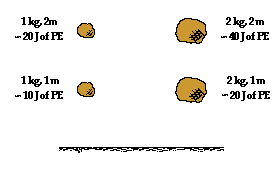vertical position above the Earth. The amount of gravitational potential energy stored in an object depended upon the amount of mass the object possessed and the amount of height to which it was raised. Gravitational potential energy depended upon object mass and object height. An object with twice the mass would have twice the potential energy and an object with twice the height would have twice the potential energy. It is common to refer to high positions as high potential energy locations. A glance at the diagram at the right reveals the fallacy of such a statement. Observe that the 1 kg mass held at a height of 2 meters has the same potential energy as a 2 kg mass held at a height of 1 meter. Potential energy depends upon more than just location; it also depends upon mass. In this sense, gravitational potential energy depends upon at least two types of quantities:

1) Mass - a property of the object experiencing the gravitational field, and

2) Height - the location within the gravitational field

So it is improper to refer to high positions within Earth's gravitational field as high potential energy positions. But is there a quantity that could be used to rate such heights as having great potential of providing large quantities of potential energy to masses that are located there? Yes! While not discussed during the unit on gravitational potential energy, it would have been possible to introduce a quantity known as gravitational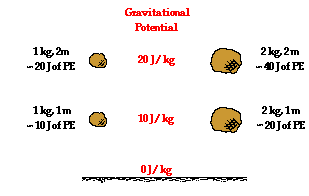potential - the potential energy per kilogram. Gravitational potential would be a quantity that could be used to rate various locations about the surface of the Earth in terms of how much potential energy each kilogram of mass would possess when placed there. The quantity of gravitational potential is defined as the PE/mass. Since both the numerator and the denominator of PE/mass are proportional to the object's mass, the expression becomes mass independent. Gravitational potential is a location-dependent quantity that is independent of the mass of the object experiencing the field. Gravitational potential describes the effects of a gravitational field upon objects that are placed at various locations within it.

If gravitational potential is a means of rating various locations within a gravitational field in terms of the amount of potential energy per unit of mass, then the concept of electric potential must have a similar meaning. Consider the electric field created by a positively charged Van de Graaff generator. The direction of the electric field is in the direction that a positive test charge would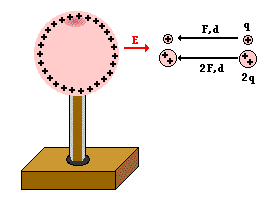be pushed; in this case, the direction is outward away from the Van de Graaff sphere. Work would be required to move a positive test charge towards the sphere against the electric field. The amount of force involved in doing the work is dependent upon the amount of charge being moved (according to Coulomb's law of electric force). The greater the charge on the test charge, the greater the repulsive force and the more work that would have to be done on it to move it the same distance. If two objects of different charge - with one being twice the charge of the other - are moved the same distance into the electric field, then the object with twice the charge would require twice the force and thus twice the amount of work. This work would change the potential energy by an amount that is equal to the amount of work done. Thus, the electric potential energy is dependent upon the amount of charge on the object experiencing the field and upon the location within the field. Just like gravitational potential energy, electric potential energy is dependent upon at least two types of quantities:

1) Electric charge - a property of the object experiencing the electrical field, and

2) Distance from source - the location within the electric field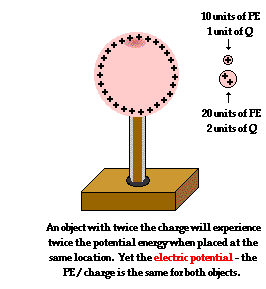While electric potential energy has a dependency upon the charge of the object experiencing the electric field, electric potential is purely location dependent. Electric potential is the potential energy per charge.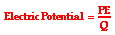The concept of electric potential is used to express the effect of an electric field of a source in terms of the location within the electric field. A test charge with twice the quantity of charge would possess twice the potential energy at a given location; yet its electric potential at that location would be the same as any other test charge. A positive test charge would be at a high electric potential when held close to a positive source charge and at a lower electric potential when held further away. In this sense, electric potential becomes simply a property of the location within an electric field. Suppose that the electric potential at a given location is 12 Joules per coulomb, then that is the electric potential of a 1 coulomb or a 2 coulomb charged object. Stating that the electric potential at a given location is 12 Joules per coulomb, would mean that a 2 coulomb object would possess 24 Joules of potential energy at that location and a 0.5 coulomb object would experience 6 Joules of potential energy at the location.

### Electric Potential in Circuits

As we begin to discuss electric circuits, we will notice that a battery powered electric circuit has locations of high and low potential.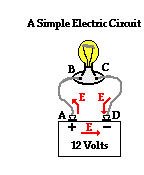Charge moving through the wires of the circuit will encounter changes in electric potential as it traverses the circuit. Within the electrochemical cells of the battery, there is an electric field established between the two terminals, directed from the positive terminal towards the negative terminal. As such, the movement of a positive test charge through the cells from the negative terminal to the positive terminal would require work, thus increasing the potential energy of every Coulomb of charge that moves along this path. This corresponds to a movement of positive charge against the electric field. It is for this reason that the positive terminal is described as the high potential terminal. Similar reasoning would lead one to conclude that the movement of positive charge through the wires from the positive terminal to the negative terminal would occur naturally. Such a movement of a positive test charge would be in the direction of the electric field and would not require work. The charge would lose potential energy as moves through the external circuit from the positive terminal to the negative terminal. The negative terminal is described as the low potential terminal. This assignment of high and low potential to the terminals of an electrochemical cell presumes the traditional convention that electric fields are based on the direction of movement of positive test charges.

In a certain sense, an electric circuit is nothing more than an energy conversion system. In the electrochemical cells of a battery-powered electric circuit, the chemical energy is used to do work on a positive test charge to move it from the low potential terminal to the high potential terminal. Chemical energy is transformed into electric potential energy within the internal circuit (i.e., the battery). Once at the high potential terminal, a positive test charge will then move through the external circuit and do work upon the light bulb or the motor or the heater coils, transforming its electric potential energy into useful forms for which the circuit was designed. The positive test charge returns to the negative terminal at a low energy and low potential, ready to repeat the cycle (or should we say circuit) all over again.

1. The quantity electric potential is defined as the amount of _____.

a. electric potential energy

b. force acting upon a charge

c. potential energy per charge

d. force per charge

2. Complete the following statement:

When work is done on a positive test charge by an external force to move it from one location to another, potential energy _________ (increases, decreases) and electric potential _________ (increases, decreases).

3. The following diagrams show an electric field (represented by arrows) and two points - labeled A and B - located within the electric field. A positive test charge is shown at point A. For each diagram, indicate whether work must be done upon the charge to move it from point A to point B. Finally, indicate the point (A or B) with the greatest electric potential energy and the greatest electric potential.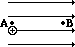Work done on charge?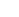YesorNo Electric PE is greatest at:AB Electric potential is greatest at:AB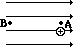Work done on charge?YesorNo Electric PE is greatest at:AB Electric potential is greatest at:AB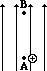Work done on charge?YesorNo Electric PE is greatest at:AB Electric potential is greatest at:AB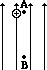Work done on charge?YesorNo Electric PE is greatest at:AB Electric potential is greatest at:AB

Next Section: